# for sample answer but values change I want 1st problem.. The figure below is a top...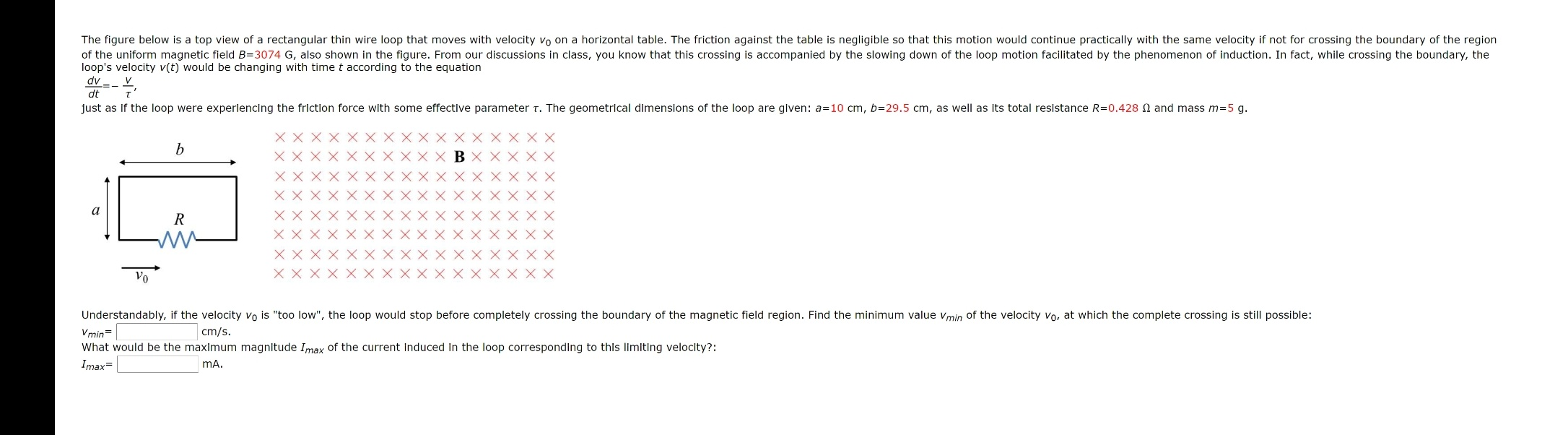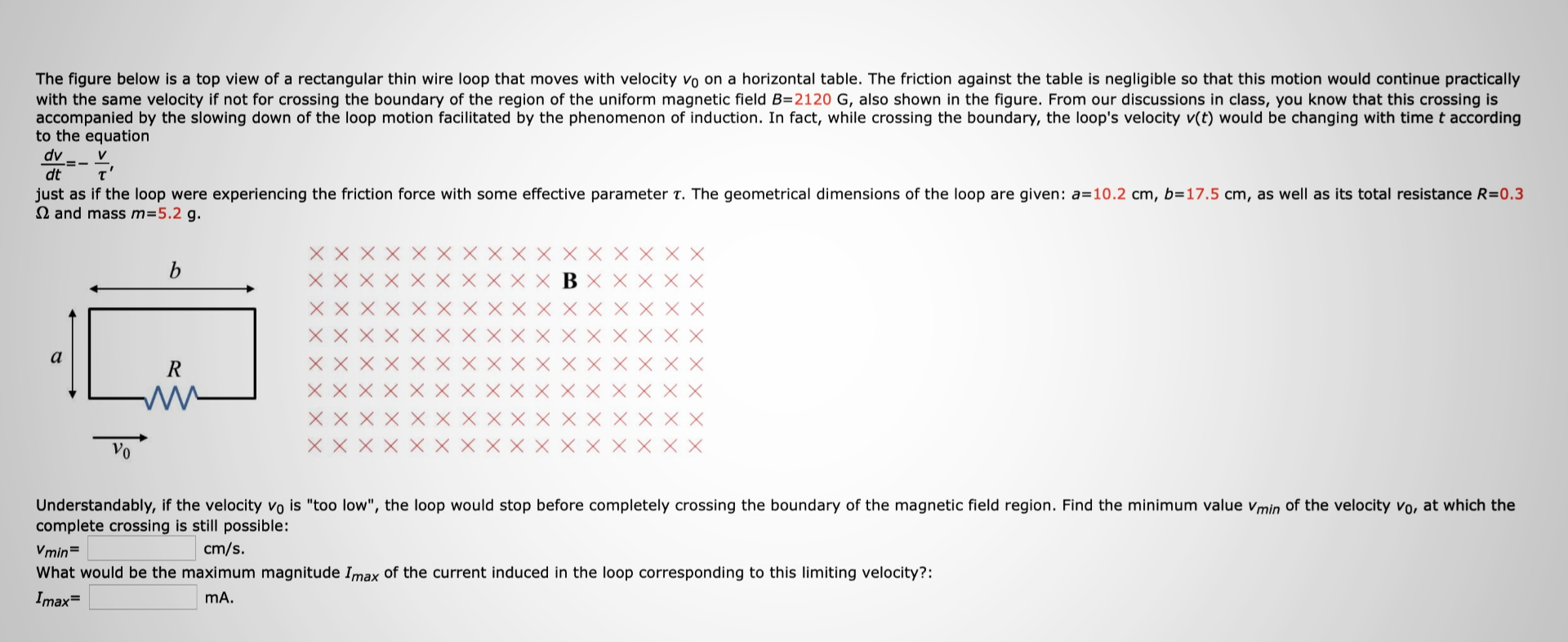for sample answer but values change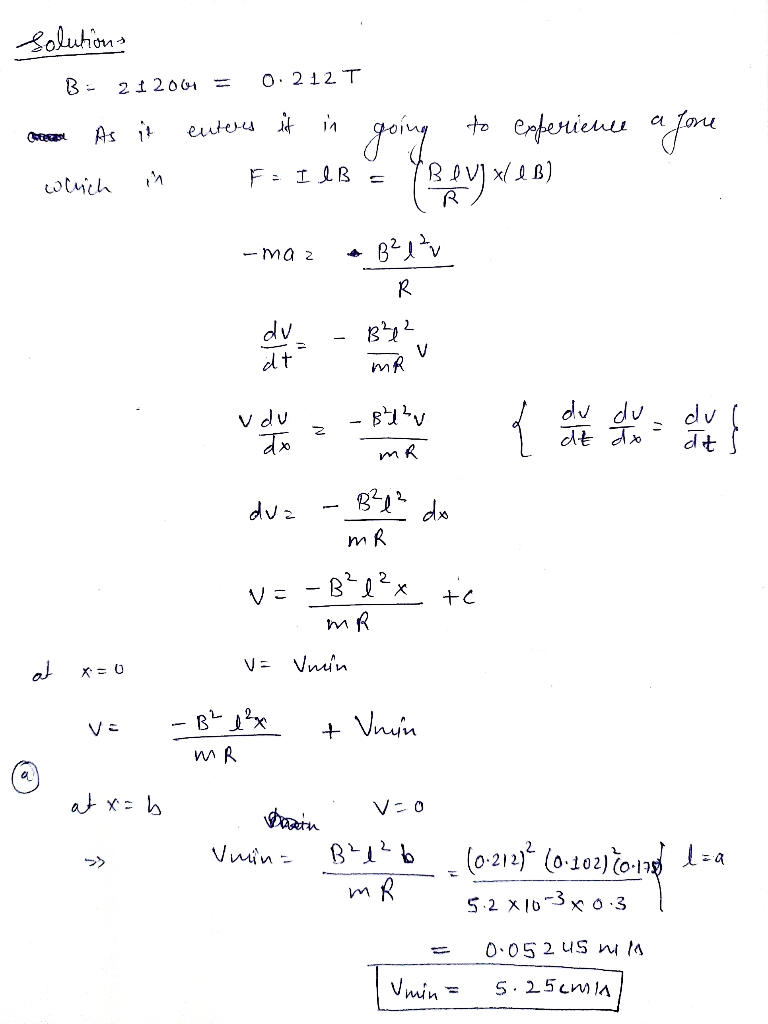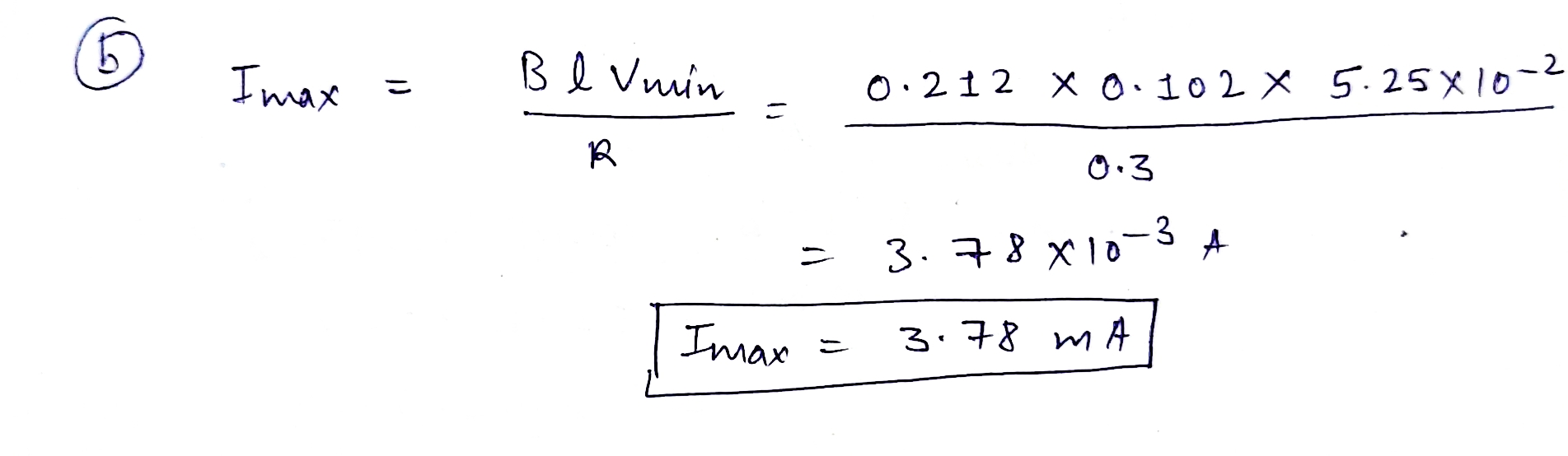I want 1st problem..

The figure below is a top view of a rectangular thin wire loop that moves with velocity vo on a horizontal table. The friction against the table is negligible so that this motion would continue practically with the same velocity if not for crossing the boundary of the region of the uniform magnetic field B=3074 G, also shown in the figure. From our discussions in class, you know that this crossing is accompanied by the slowing down of the loop motion facilitated by the phenomenon of induction. In fact, while crossing the boundary, the loop's velocity v(t) would be changing with time t according to the equation dv - у dt т" just as if the loop were experiencing the friction force with some effective parameter t. The geometrical dimensions of the loop are given: a=10 cm, b=29.5 cm, as well as its total resistance R=0.428 I and mass m=5 g. b ХХХХХХХХХХ ХХХХ ХХХХ Ххх в ХХХХ ХХХХХХХХХХХХХХХХ ХХХХХХХХХХХХХХХХ xxxx ХХХХХ ХХХХ xxx XXX ХХХХ ХХХХХ а R M vo Understandably, if the velocity vo is "too low", the loop would stop before completely crossing the boundary of the magnetic field region. Find the minimum value V min of the velocity vo, at which the complete crossing is still possible: Vmin= cm/s. What would be the maximum magnitude Imax of the current induced in the loop corresponding to this limiting velocity?: Imax= mA.
The figure below is a top view of a rectangular thin wire loop that moves with velocity vo on a horizontal table. The friction against the table is negligible so that this motion would continue practically with the same velocity if not for crossing the boundary of the region of the uniform magnetic field B=2120 G, also shown in the figure. From our discussions in class, you know that this crossing is accompanied by the slowing down of the loop motion facilitated by the phenomenon of induction. In fact, while crossing the boundary, the loop's velocity v(t) would be changing with time t according to the equation dv V dt just as if the loop were experiencing the friction force with some effective parameter t. The geometrical dimensions of the loop are given: a=10.2 cm, b=17.5 cm, as well as its total resistance R=0.3 12 and mass m=5.2 g. 늘 b xxxxxxxxx XXX ХХХХХХХХХХ В XX ХХХХХХХХХХХХХХХХ ХХХХХХХХХХХХХХХХ ХХХХХХХХ XXX XX ХХХХХХ XXX ХХХХХХХХХ XXX a R Vo Understandably, if the velocity vo is "too low", the loop would stop before completely crossing the boundary of the magnetic field region. Find the minimum value Vmin of the velocity vo, at which the complete crossing is still possible: Vmin= cm/s. What would be the maximum magnitude Imax of the current induced in the loop corresponding to this limiting velocity?: Imax= mA.
solutions BE 21206 0.212T As it enters it in which in F: IIB xl e B) going to experience a Jone Roy B2lv ma 2 R du dt B²12 MR V v du atx 82v MR re { che la de la du B²1² do duz mR Va -B?l?x MR tc at VE Vnin x=0 • B² 1² MR + Vnin at x=b V=0 Pasin V min - B²1²b + (0-212] (0.102) 70-178 1za del comune mR 5.2 x 103 x 0.3 0.052 US mily 5.25cmia Umin
Imax B l Vimin = 0.212 x 0.102 * 5.25X10-2 li R 0.3 11 3.78X10-3 A Imax 3.78 m A

Please refer to the below images for detailed explanation: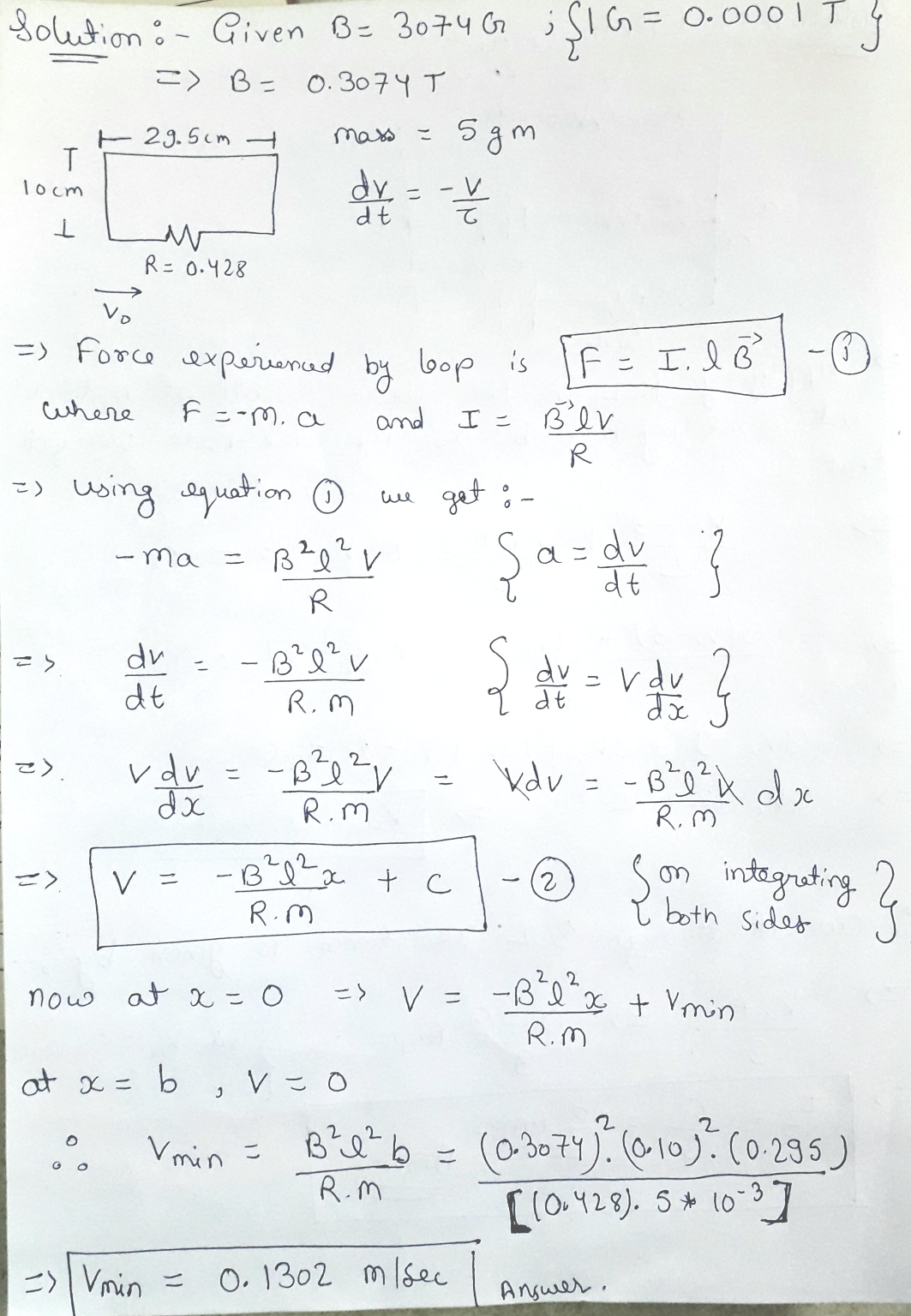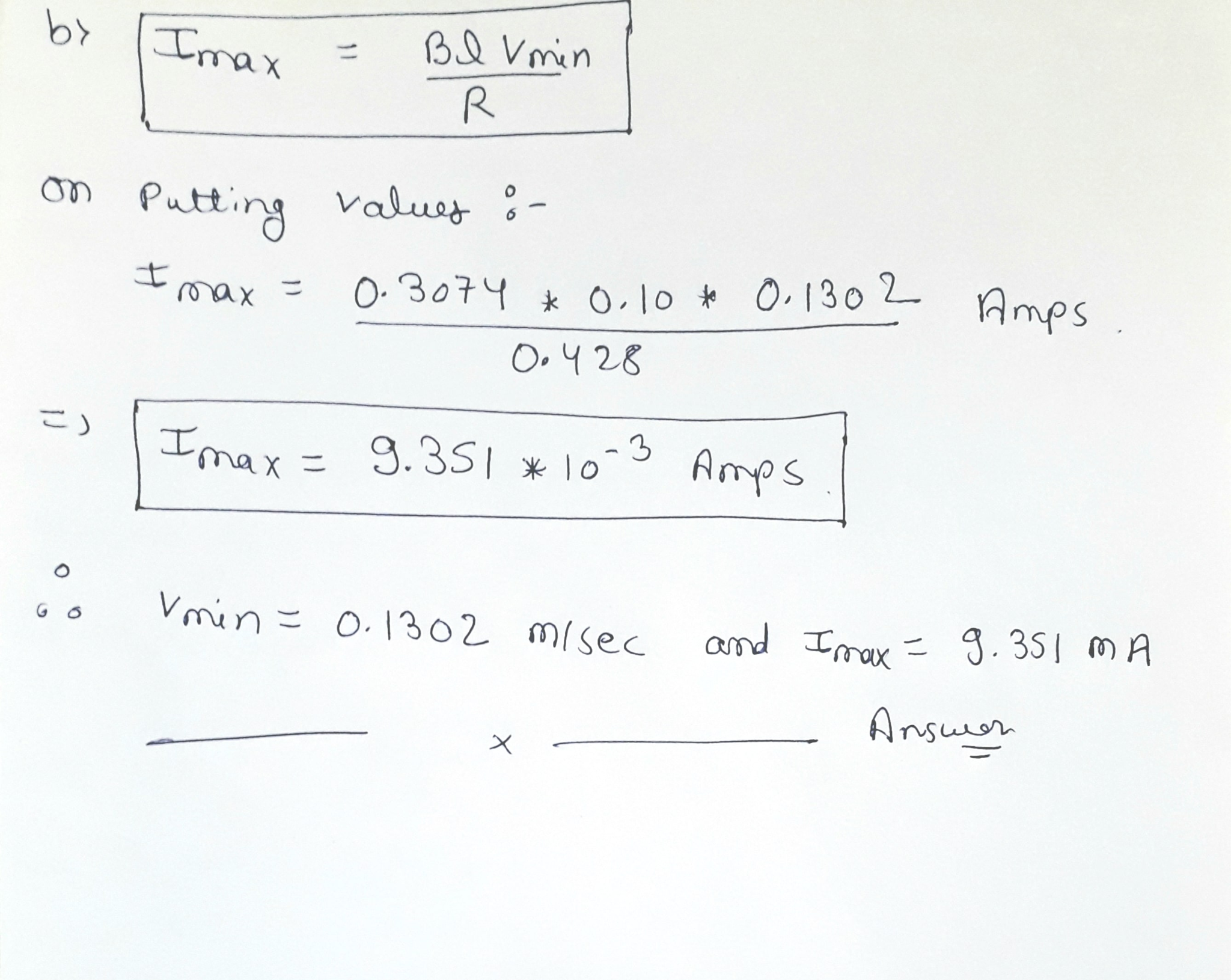##### Add Answer of: for sample answer but values change I want 1st problem.. The figure below is a top...
Similar Homework Help Questions
• ### The figure below is a top view of a rectangular thin wire loop that moves with...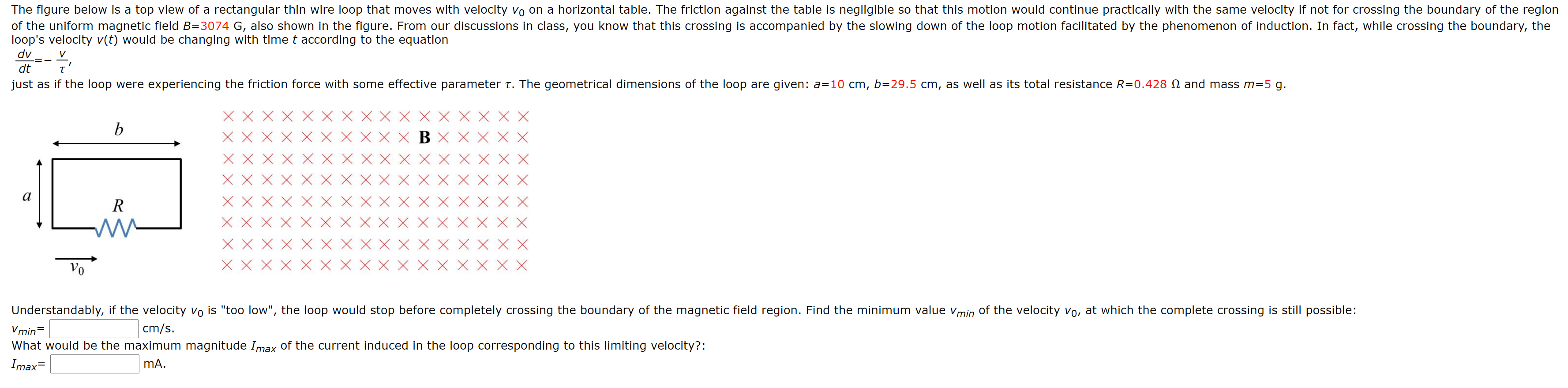The figure below is a top view of a rectangular thin wire loop that moves with velocity vo on a horizontal table. The friction against the table is negligible so that this motion would continue practically with the same velocity if not for crossing the boundary of the region of the uniform magnetic field B=3074 G, also shown in the figure. From our discussions in class, you know that this crossing is accompanied by the slowing down of the loop...

• ### A very long, rectangular loop of wire can slide without friction on a horizontal surface. Initially...A very long, rectangular loop of wire can slide without friction on a horizontal surface. Initially the loop has part of its area in a region of uniform magnetic field that has magnitude B = 3.30 T and is perpendicular to the plane of the loop. The loop has dimensions 4.00 cm by 60.0 cm, mass 26.0 g , and resistance R = 7.00×10−3 Ω . The loop is initially at rest; then a constant force Fext = 0.180 N...

• ### How do you do this? 7. -9 points My Notes Ask Your Teacher The figure below...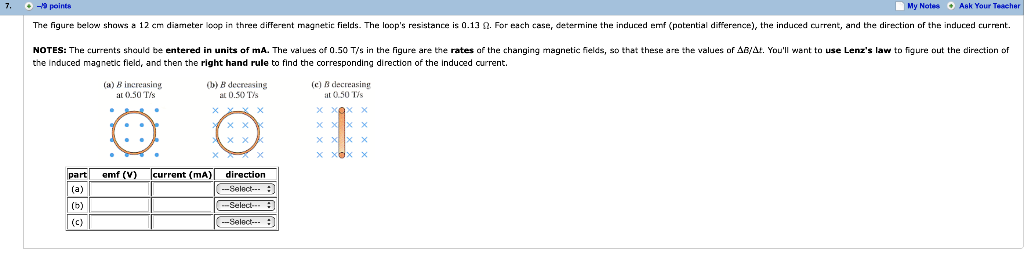How do you do this? 7. -9 points My Notes Ask Your Teacher The figure below shaws a 12 cm diameter loop in three different magnetic fields. The loop's resistance is 0.13 2. For each case, determine the induced emf (potential difference), the induced current, and the direction of the induced current. NOTES: The currents shauld be entered in units of mA. The values of 0.50 T s in the fiaure are the rates af the changing magnetic fields, so...

• ### 1. Asteady, uniform magnetic field of magnitude B exists in the horizontal, shaded region. This field is directed downw...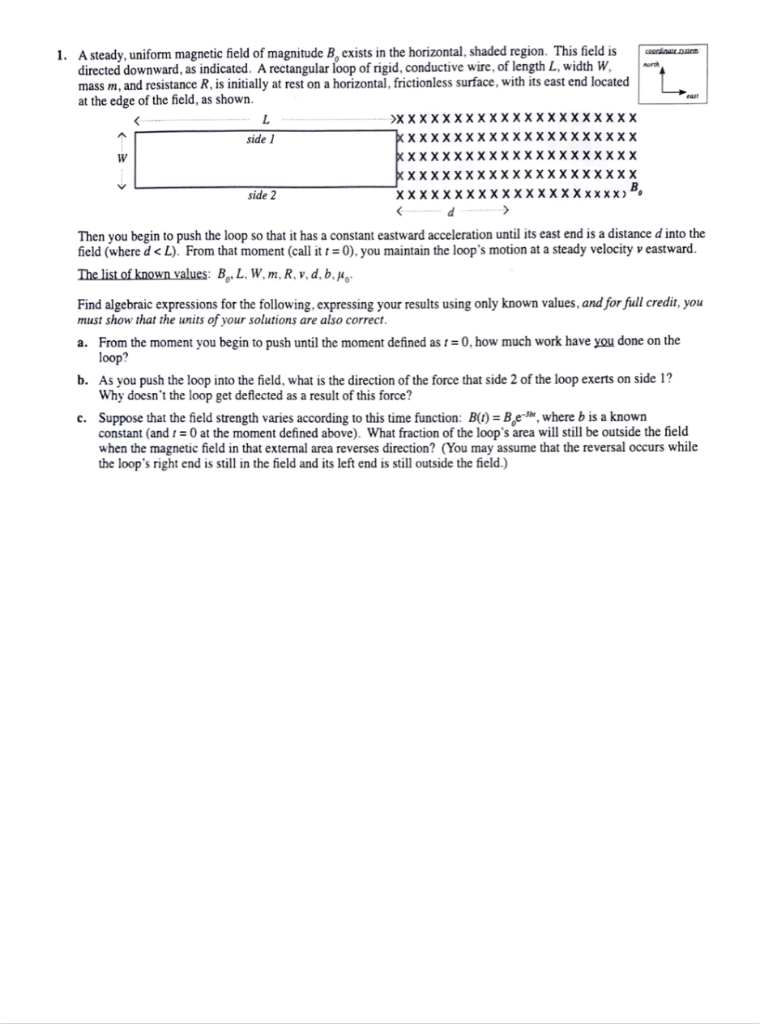1. Asteady, uniform magnetic field of magnitude B exists in the horizontal, shaded region. This field is directed downward, as indicated. A rectangular loop of rigid, conductive wire, of length L, width W mass m, and resistance R, is initially at rest on a horizontal, frictionless surface, with its east end located at the edge of the field, as shown. CAardnae em >XXXXX XXXXXX XXXXXXXXXX XXX XXXXXXX XX XXXXXXX. side 1 КХXXXX XXXXXXХ W XXXXXXX ХXXXXXXXXXXXX K XX) XXXXXXX XXXXXXXXXXXXX>"...

• ### Will give an up vote only if all parts are answered and detailed explanations are given....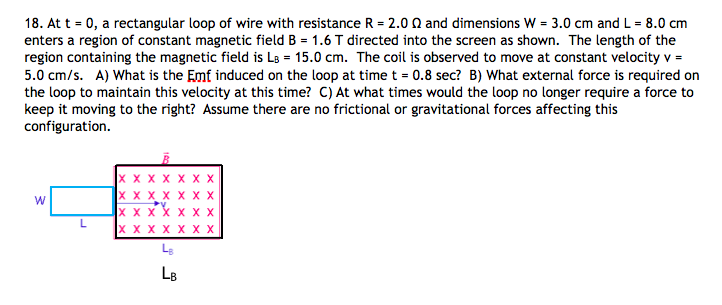Will give an up vote only if all parts are answered and detailed explanations are given. Thank you. 18. At t 0, a rectangular loop of wire with resistance R = 2.0 0 and dimensions W 3.0 cm and L 8.0 cm enters a region of constant magnetic field B 1.6 T directed into the screen as shown. The length of the region containing the magnetic field is La 15.0 cm. The coil is observed to move at constant velocity...

• ### Thank you Very Much! I just want to check my answers before I turn this in!...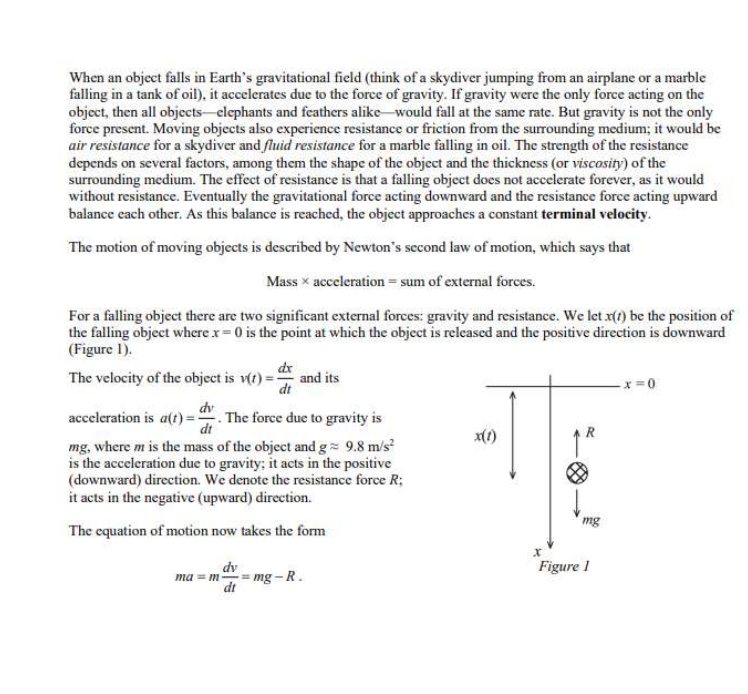Thank you Very Much! I just want to check my answers before I turn this in! Would you be able to type this answer out? When an object falls in Earth's gravitational field (think of a skydiver jumping from an airplane or a marble falling in a tank of oil), it accelerates due to the force of gravity. If gravity were the only force acting on the object, then all objects-elephants and feathers alike would fall at the same rate....

• ### need before 12:00 noon central time july 30, 2020 Problem 3 A particle with charge of...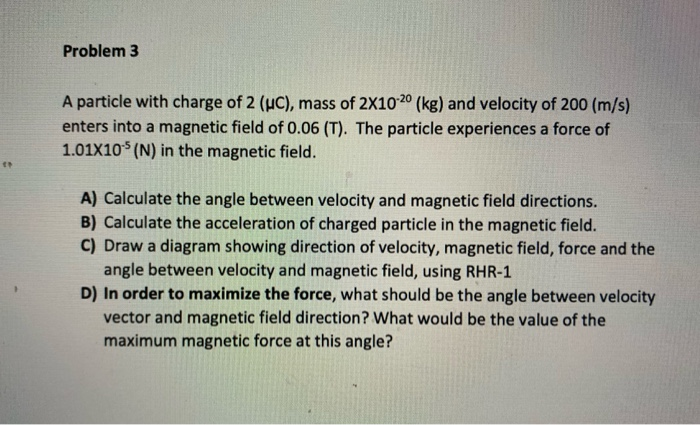need before 12:00 noon central time july 30, 2020 Problem 3 A particle with charge of 2 (uc), mass of 2X10-20 (kg) and velocity of 200 (m/s) enters into a magnetic field of 0.06 (T). The particle experiences a force of 1.01X10% (N) in the magnetic field. A) Calculate the angle between velocity and magnetic field directions. B) Calculate the acceleration of charged particle in the magnetic field. C) Draw a diagram showing direction of velocity, magnetic field, force and...

• ### 1. A steady, uniform magnetic field of magnitude B, exists in the horizontal, shaded region. This field is directed...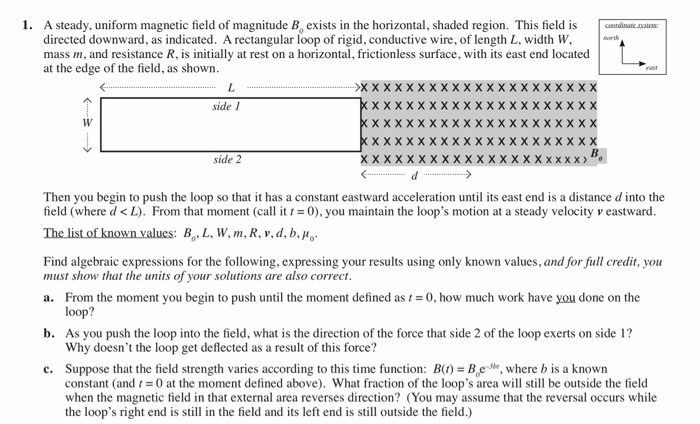1. A steady, uniform magnetic field of magnitude B, exists in the horizontal, shaded region. This field is directed downward, as indicated. A rectangular loop of rigid, conductive wire, of length L, width W mass m, and resistance R, is initially at rest on a horizontal, frictionless surface, with its east end located at the edge of the field, as shown couninate natn north L easr side 1 X X X X W X X side 2 X X d...

• ### easy problem just cant figure it out i think a formula is the answer just can figure out which...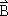A metal wire of mass m slides without friction on twohorizontal rails spaced a distance d apart, as in thefigure below. The track lies in a verticaluniform magnetic field. Generator G produces a constant current iin the wire andthe rails (even as the wire moves). Find thevelocity of the wire's motion as a function of time, assuming it tobe stationary at t = 0. (Use m,d,B, i and t as necessary, where allquantities are in SI units. Enter the sign...

• ### Question 7 The conducting rod shown in the figure has length L and is being pulled along horlizontal, frictionless, conducting rails at a constant metal strip. A uniform magnetic field, directed...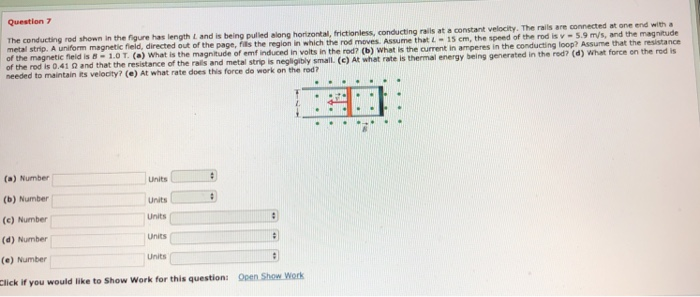Question 7 The conducting rod shown in the figure has length L and is being pulled along horlizontal, frictionless, conducting rails at a constant metal strip. A uniform magnetic field, directed of the magnetic fieid is 8-1.0 T. (a) What is the magnitude Assume that L15 cm, the speed of the rod is v -5.9 m/s, and the magnitude of emf induced in voits in the rod? (b) What is the current in amperes in the conducting loop? Assume that...

Free Homework App